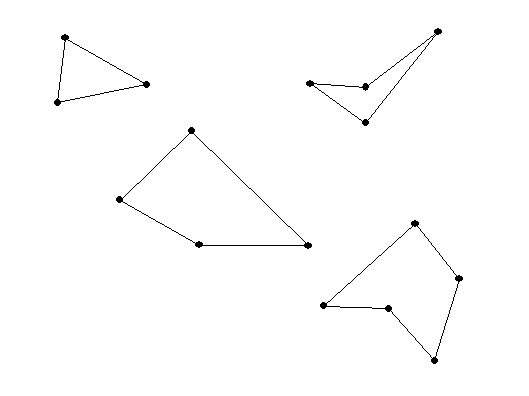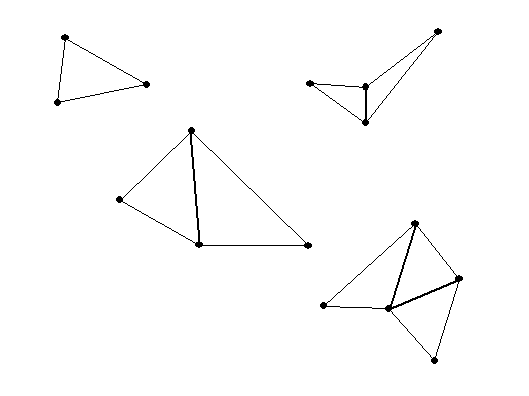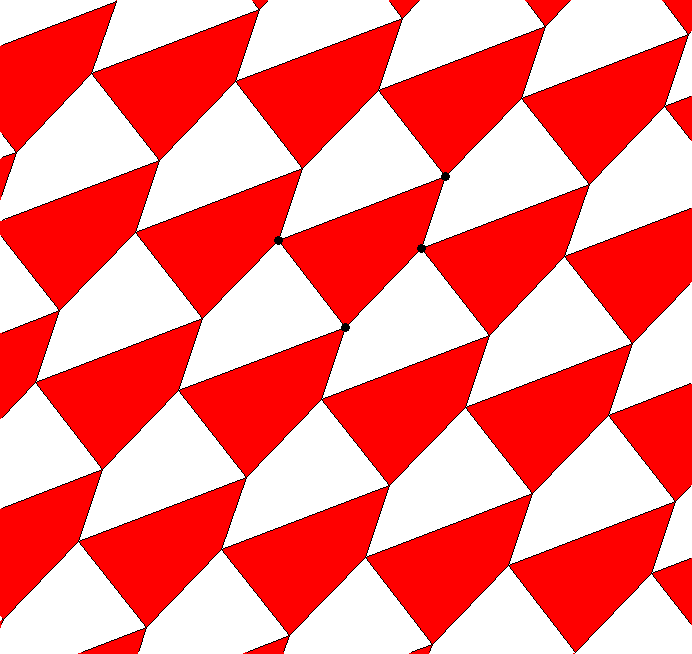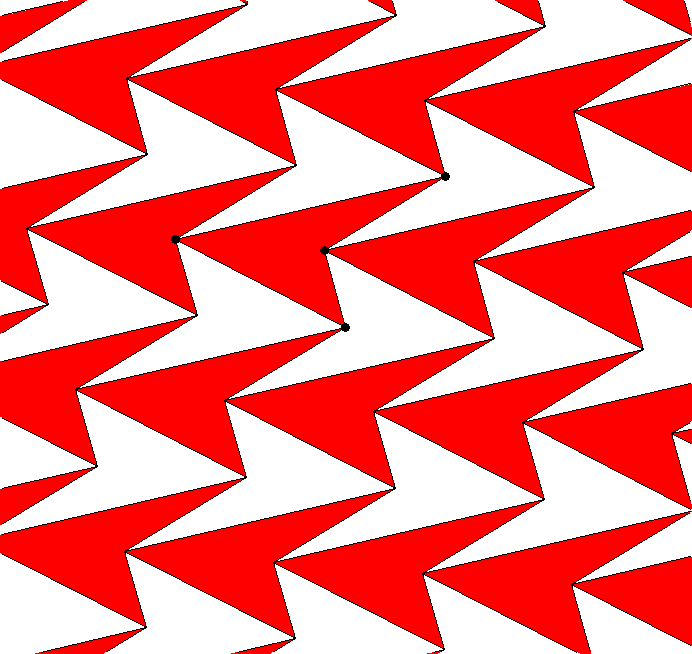Monday, February 10, 2014

Class #6

• Example: Numbers for counting and measuring...
• numeral: a symbol for representing a number
• Number: a form of universal language to describe anything/ physical things/ concepts related to measurement
• Numerals : such as V, 5, five, cinq, chamesh, cinco

Geometric Puzzle Foundations
• Measuring angles, lengths and areas.
• Triangles  : add to 180 degrees- straight angle [Illustrate physically and with Wingeometry andGeoGebra]
• Squares, rectangles  : 90 degree/ right angle

More on measurements of angles of polygons with n sides. .
When n = 3 this is a triangle, n=4, a quadrilateral, or when n= 5, a pentagon.The sum of the measures of the interior angles of a triangle is 180 degrees.
What about a quadrilateral? and a pentagon?  or an n sided polygon  ( an "n -gon")?••